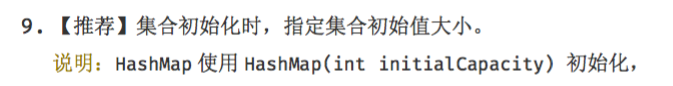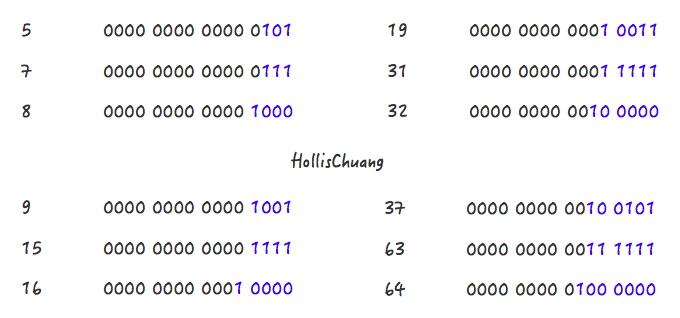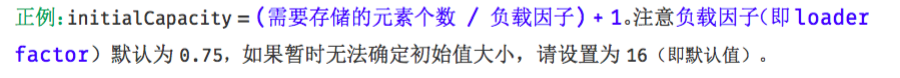Hollis出品的全套Java面试宝典不来了解一下吗？

# 关于HashMap容量的初始化，还有这么多学问。

Hollis出品的全套Java面试宝典不来了解一下吗？

### 为什么要设置HashMap的初始化容量``````public static void main(String[] args) {
int aHundredMillion = 10000000;

Map<Integer, Integer> map = new HashMap<>();

long s1 = System.currentTimeMillis();
for (int i = 0; i < aHundredMillion; i++) {
map.put(i, i);
}
long s2 = System.currentTimeMillis();

System.out.println("未初始化容量，耗时 ： " + (s2 - s1));

Map<Integer, Integer> map1 = new HashMap<>(aHundredMillion / 2);

long s5 = System.currentTimeMillis();
for (int i = 0; i < aHundredMillion; i++) {
map1.put(i, i);
}
long s6 = System.currentTimeMillis();

System.out.println("初始化容量5000000，耗时 ： " + (s6 - s5));

Map<Integer, Integer> map2 = new HashMap<>(aHundredMillion);

long s3 = System.currentTimeMillis();
for (int i = 0; i < aHundredMillion; i++) {
map2.put(i, i);
}
long s4 = System.currentTimeMillis();

System.out.println("初始化容量为10000000，耗时 ： " + (s4 - s3));
}
``````

``````未初始化容量，耗时 ： 14419

``````

### HashMap中容量的初始化

``````Map<String, String> map = new HashMap<String, String>(1);
map.put("hahaha", "hollischuang");

Class<?> mapType = map.getClass();
Method capacity = mapType.getDeclaredMethod("capacity");
capacity.setAccessible(true);
System.out.println("capacity : " + capacity.invoke(map));
``````

``````    int n = cap - 1;
n |= n >>> 1;
n |= n >>> 2;
n |= n >>> 4;
n |= n >>> 8;
n |= n >>> 16;
return (n < 0) ? 1 : (n >= MAXIMUM_CAPACITY) ? MAXIMUM_CAPACITY : n + 1;
``````Step 1，5->7

Step 2，7->8

Step 1，9->15

Step 2，15->16

Step 1，19->31

Step 2，31->32

``````n |= n >>> 1;
n |= n >>> 2;
n |= n >>> 4;
n |= n >>> 8;
n |= n >>> 16;
``````

``````return (n < 0) ? 1 : (n >= MAXIMUM_CAPACITY) ? MAXIMUM_CAPACITY : n + 1;
``````

Step 2 比较简单，就是做一下极限值的判断，然后把Step 1得到的数值+1。

Step 1 怎么理解呢？其实是对一个二进制数依次向右移位，然后与原值取或。其目的对于一个数字的二进制，从第一个不为0的位开始，把后面的所有位都设置成1。

``````1100 1100 1100 >>>1 = 0110 0110 0110
1100 1100 1100 | 0110 0110 0110 = 1110 1110 1110
1110 1110 1110 >>>2 = 0011 1011 1011
1110 1110 1110 | 0011 1011 1011 = 1111 1111 1111
1111 1111 1111 >>>4 = 1111 1111 1111
1111 1111 1111 | 1111 1111 1111 = 1111 1111 1111
``````

``````Step 1:
0100 >>>1 = 0010
0100 | 0010 = 0110
0110 >>>1 = 0011
0110 | 0011 = 0111
Step 2:
0111 + 0001 = 1000
``````

``````int n = cap - 1;
``````

``````    int n = cap - 1;
n |= n >>> 1;
n |= n >>> 2;
n |= n >>> 4;
n |= n >>> 8;
n |= n >>> 16;
return (n < 0) ? 1 : (n >= MAXIMUM_CAPACITY) ? MAXIMUM_CAPACITY : n + 1;
``````

### HashMap中初始容量的合理值``````public static <K, V> HashMap<K, V> newHashMapWithExpectedSize(int expectedSize) {
return new HashMap<K, V>(capacity(expectedSize));
}

/**
* Returns a capacity that is sufficient to keep the map from being resized as long as it grows no
* larger than expectedSize and the load factor is ≥ its default (0.75).
*/
static int capacity(int expectedSize) {
if (expectedSize < 3) {
checkNonnegative(expectedSize, "expectedSize");
return expectedSize + 1;
}
if (expectedSize < Ints.MAX_POWER_OF_TWO) {
// This is the calculation used in JDK8 to resize when a putAll
// happens; it seems to be the most conservative calculation we
// can make.  0.75 is the default load factor.
return (int) ((float) expectedSize / 0.75F + 1.0F);
}
return Integer.MAX_VALUE; // any large value
}
``````

`return (int) ((float) expectedSize / 0.75F + 1.0F);`上面有一行注释，说明了这个公式也不是guava原创，参考的是JDK8中putAll方法中的实现的。感兴趣的读者可以去看下putAll方法的实现，也是以上的这个公式。

### 总结

``````Map<String, String> map = Maps.newHashMapWithExpectedSize(10);
``````

Hollis出品的全套Java面试宝典不来了解一下吗？

### 评论 4

1. #1

思考题答案，我觉得是因为putAll 是已知要放多少个元素。对吗

闲云清烟6年前 (2018-06-20)回复
2. #2

写的很详细，通俗易懂。
发现几处小笔误，首先aHundredMillion设置成了一千万，而不是一亿。
然后，“但是还有一种特殊情况套用以上公式不行，这些数字就是2的幂自身。如果数字4 套用公式的话。得到的会是 8 ：”下面的代码第二次应该是右移两位取或吧？不过不影响最终结果

AnoLz5年前 (2019-03-27)回复
3. #3

其实当不确定put值大小的时候 有时间和空间的取舍关系

boolean5年前 (2019-05-27)回复
4. #4

`把默认容量设置成expectedSize / 0.75F + 1.0F 是一个在性能上相对好的选择，但是，同时也会牺牲些内存`
把默认容量设置成小一点值，比如expectedSize，放入数据后也会扩容到上面的容量呀，感觉没有`牺牲些内存`一说

NotMeBug3年前 (2021-03-05)回复